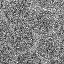# Theory

The CTF's used in various software packages and given in many textbooks vary slightly but significantly. It is therefore important that the determination and application of CTF parameters be done with the same package. In Bsoft, the following version of the CTF at spatial frequency s is used: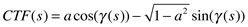where a is the amplitude contribution and the phase is given by: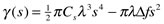where Cs is the spherical aberration, lambda is the electron wavelength (derived from the accelerating voltage), and the defocus is given in terms of the average and deviation, or maximum and minimum, by: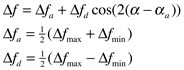where alpha(a) is the astigmatism angle.

The reciprocal space distance is:

s = sqrt(x^2 + y^2)

The electron wavelength is: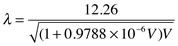The CTF is now recast into the full aberration terms.

# Examples using bctf

 A fan pattern with all spatial frequencies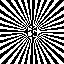Part of a micrograph